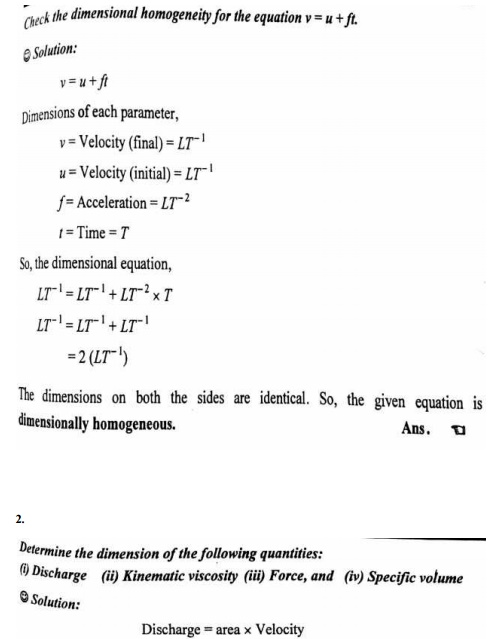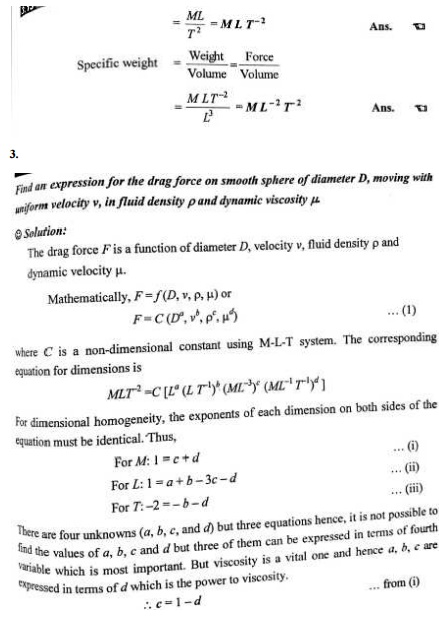Home | | Fluid Mechanics and Machinery | Important Answers and Solved Problems: Fluid Flow Through Circular Conduits

# Important Answers and Solved Problems: Fluid Flow Through Circular Conduits

Mechanical - Fluid Mechanics And Machinery - Dimensional Analysis

DIMENSIONAL ANALYSIS

1. What are the methods of dimensional analysis

There are two methods of dimensional analysis.  They are,

a. Rayleigh - Retz method

b. Buckingham's   theotem method.

Nowadays Buckingham's   theorem method is only used.

2. Describe the Rayleigh's method for dimensional analysis.

Rayleigh's method is used for determining the expression for a variable which depends upon maximum three or four variables only. If the number of independent variables becomes more than four, then it is very difficult to find the expression for dependent variable.

3. What do you mean by dimensionless number

Dimensionless numbers are those numbers which are obtained by dividing the inertia force by viscous force or gravity force or pressure force or surface tension or elastic force. As this is a ratio of one force to other force, it will be a dimensionless number.

4.  Name the different forces present in fluid flow

Inertia force Viscous force Surface tension force Gravity force

5.  State Buckinghams Π theorem

It states that if there are ‘n’ variables in a dimensionally homogeneous equation and if these variables contain m fundamental dimensions (M,L,T), then they are grouped into (n-m), dimensionless independent Π-terms.

6. State the limitations of dimensional analysis.

1. Dimensional analysis does not give any due regarding the selection of variables. 2.The complete information is not provided by dimensional analysis.

3.The values of coefficient and the nature of function can be obtained only by experiments or from mathematical analysis.

7.Define Similitude

Similitude is defined as the complete similarity between the model and prototype.

8. State Froude’s model law

Only Gravitational force is more predominant force. The law states ‘The Froude’s number is same for both model and prototype’

9.What are the similarities between model and prototype?

(i)                Geometric Similarity

(ii)             Kinematicc Similarity

(iii)           Dynamic Similarity

10.Define Weber number.

It is the ratio of the square root of the inertia force to the surface tension force.Study Material, Lecturing Notes, Assignment, Reference, Wiki description explanation, brief detail
Mechanical - Fluid Mechanics And Machinery - Dimensional Analysis : Important Answers and Solved Problems: Fluid Flow Through Circular Conduits |

Related Topics